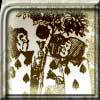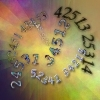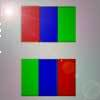#### You may also like### Painting Cubes

Imagine you have six different colours of paint. You paint a cube using a different colour for each of the six faces. How many different cubes can be painted using the same set of six colours?### Permute It

Take the numbers 1, 2, 3, 4 and 5 and imagine them written down in every possible order to give 5 digit numbers. Find the sum of the resulting numbers.### Flagging

How many tricolour flags are possible with 5 available colours such that two adjacent stripes must NOT be the same colour. What about 256 colours?

# And So on and So On

##### Age 11 to 14 Challenge Level:

There were lots of correct solutions to this problem and many different methods were employed.

Below are three of them.

Chris, from St Bees School in Cumbria offered two methods:

First Method

(this method was also suggested by Andrei (from School 205, Bucharest) and Atharv ( from Bedford Modern School), both of whom listed all the numbers.

We observe there are 4!  different numbers.

We can add these on their own

2457 2475 2547 2574 2745 2754
4257 4275 4527 4572 4725 4752
5247 5274 5427 5472 5724 5742
7245 7254 7425 7452 7524 7542

Second Method

We observe there are 4!  different numbers.

We can observe that these are symmetrical around the mean (editor's comment: Why?) and so will add to:

1/2[S + L]n

S = smallest number, L= largest number, n=total numbers [4! here]

Both methods give the solution as 119988

Third Method

By Langran-Goldsmith, Rebecca, Caroline and Gemma (from Northampton High School). A similar method was used by Clement.

Each digit appears six times in each position.

So you need to add together:

6 x (2000 + 4000 + 5000 + 7000) + 6 x (200 + 400 + 500 + 700) + 6 x (20 + 40 + 50 + 70) + 6 x (2 + 4 + 5 + 7) = 119988.